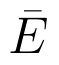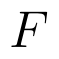Select Page

# CBSE Maths 12 Science Probability MCQ Answers in English

CBSE Maths 12 Science Probability MCQ Answers in English to enable students to get Answers in a narrative video format for the specific question.

Expert Teacher provides CBSE Maths 12 Science Probability MCQ Answers through Video Answers in English language. This video solution will be useful for students to understand how to write an answer in exam in order to score more marks. This teacher uses a narrative style for a question from Probability not only to explain the proper method of answering question, but deriving right answer too.

Please find the question below and view the Answer in a narrative video format.

Question:

## Similar Questions from CBSE, 12th Science, Maths, Probability

Question 1 : In a game, a man wins rupee five for number six and losses rupee one for any other number, when a fair die is thrown. The man decided to throw a die thrice but to quit as and when he gets a six. Find the expected value of the amount he wins/losses. (View Answer Video)

Question 3 :  If E and F are independent events, then show thatandare also independent.  (View Answer Video)

Question 4 : Three cards are drawn without replacement from a pack of 52 cards. Find the probability that the cards drawn are king, queen and jack respectively.

Question 5 : A card from a pack of 52 cards is lost. From the remaining cards of the pack, two cards are drawn at random and are found to be hearts. Find the probability of the missing card to be a heart.     (View Answer Video)

### Differential Equations

Question 1 : Write the differential equation representing the family of curves y = mx, where m is an arbitrary constant. (View Answer Video)

Question 2 : Find the particular solution of the differential equationgiven that y = 0, when x = 0. (View Answer Video)

Question 3 : Solve the differential equationgiven that y = 1 when x = 1. (View Answer Video)

Question 4 : Find the particular solution of the differential equationgiven that y = 1, when x = 0. (View Answer Video)

Question 5 : Find the particular solution of the differential equation :when x = 1,(View Answer Video)

### Application of Integrals

Question 1 : Find the area of the given curves and given lines:and x-axis (View Answer Video)

Question 2 : Find the area of the smaller region bounded by the ellipseand the straight line 8x + 3y = 12. (View Answer Video)

Question 3 : Find the area bounded by curves. (View Answer Video)

Question 4 : Find the area enclosed by the parabolaand the line x - y = 4. (View Answer Video)

Question 5 : Find the area of the region bounded by the curveand the line x = 3. (View Answer Video)

### Integrals

Question 1 : Find :(View Answer Video)

Question 2 : Evaluate :(View Answer Video)

Question 3 : Evaluate :(View Answer Video)

Question 4 : Find. (View Answer Video)

Question 5 :(View Answer Video)